### Home > MC2 > Chapter 2 > Lesson 2.2.3 > Problem2-71

2-71.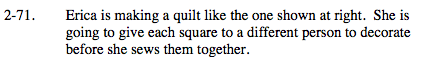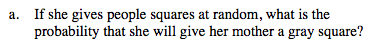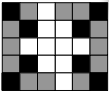You can write probability using a fraction:

$\frac{\# \text{ of squares we're looking for}}{\# \text{ of total squares}}$

Now count the number of gray squares, then the number of total squares to get the probability.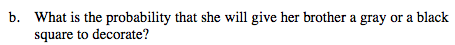Refer to part (a) for help.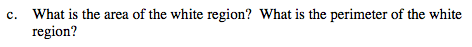To find the area, count the number of squares since each is one square unit. To find the perimeter, use the toothpick method you learned in chapter one.

Area = 10 square units
Perimeter = 18 units